﻿ 点蚀损伤钢板在空爆冲击波载荷作用下的动态响应研究
 舰船科学技术2018, Vol. 40Issue (4): 27-32,38PDF

1. 海军驻上海江南造船（集团）有限责任公司军事代表室，上海 201913;
2. 海军工程大学 舰船工程系，湖北 武汉 430033

Response of corroded steel plates due to air blast load
WANG Jin1, DENG Xian-hui1, WU Fan2
1. Naval Military Representative Office in Jiangnan Shipyard (Group) Co., Ltd., Shanghai 201913, China;
2. Department of Naval Architecture Engineering, Naval University of Engineering, Wuhan 430033, China
Abstract: The main objective of this study is to explore the dynamic response of a clamped square steel plate with corrosion pits under the air blast loads. Through finite element program Ansys/LS-DYNA, simulations of the response behavior of the corroded plate subjected to the blast loading are conducted. The effects of influential parameters including, degree of pit, pit depth, and different corrosion patterns at each side of the plate, in both-sided corroded plates. The results show that by increasing degree of pitting and depth of pits can cause the most reduction of dynamic load carrying capacity, whereas position of pitted surface in respect to applied loading affects the dynamic load carrying capacity of corroded plates insignificantly.
Key words: air blast loads     corroded steel plate     dynamic response     numerical simulation
0 引　言

TSCF根据点蚀损伤钢板的弯曲试验，理论推导了一个包含弯曲刚度、质量、边界条件和尺寸等参数的点蚀损伤钢板等效厚度计算公式。Daidora提出了一种用于估计结构腐蚀后剩余厚度的数学方法。Flaks，Paik等开展了点蚀损伤板构件及老龄船舶极限强度的研究。Dunbar，Nakai等开展了点蚀损伤对船舶典型构件局部强度的影响研究。Huang采用非线性有限元软件（FEM）研究了点蚀损伤板的极限强度。徐强建立了点蚀损伤壳板的力学模型，以超参数壳元为基础推导了坑点腐蚀壳体单元，并以坑点腐蚀壳体单元为基础开展了算例分析。徐强分别采用PCSE、基于MPC的Shell-Solid集成以及Solid三种有限元方法对含点蚀损伤的深海耐压球壳的强度和稳定性进行了对比计算。Rahbar-Ranji运用FEM研究了含有不规则表面的腐蚀损伤板的极限强度、塑性断裂强度和抗弯强度。

1 数值计算模型

 $P(\tau ) = {P_{{r}}} \cdot {\cos ^2}\theta + {P_{{i}}} \cdot (1 + {\cos ^2}\theta - 2\cos \theta )\text{，}$ (1)

 ${\sigma _{{d}}} = \left( {{\sigma _0} + \beta \frac{{E{E_{\tan }}}}{{E - {E_{\tan }}}}{\varepsilon _{{p}}}} \right)\left[ {1 + {{\left( {\frac{{\dot \varepsilon }}{C}} \right)}^{1/n}}} \right]\text{，}$ (2)表 1 钢板的材料参数 Tab.1 Material parameters of plate
2 数值计算结果与分析 2.1 数值计算方法验证

 $\begin{split}& \frac{{3\sqrt 3 {\pi ^2}\left( {{a^2} + {b^2}} \right) + 256ab}}{{32\sqrt 3 ab}}h{\sigma _s}{\omega _0}^2 + \\& \quad \quad \frac{{\sqrt 3 \pi \left( {{a^2} \!\!+\!\! {b^2}} \right) \!\!+\!\! 8ab}}{{2\sqrt 3 ab}}{h^2}{\sigma _s}{\omega _0} \!\!=\!\! 8\frac{{ab{A_i}^2}}{{\rho h}}{Q^{4/3}}{r^{ - 2}}\text{。}\end{split}$ (3)表 2 单元尺寸对数值计算结果的影响 Tab.2 Mesh sensitivity analysis showing the predicted maximum deflection (mm) of an uncorroded plate (0.5 m×0.5 m)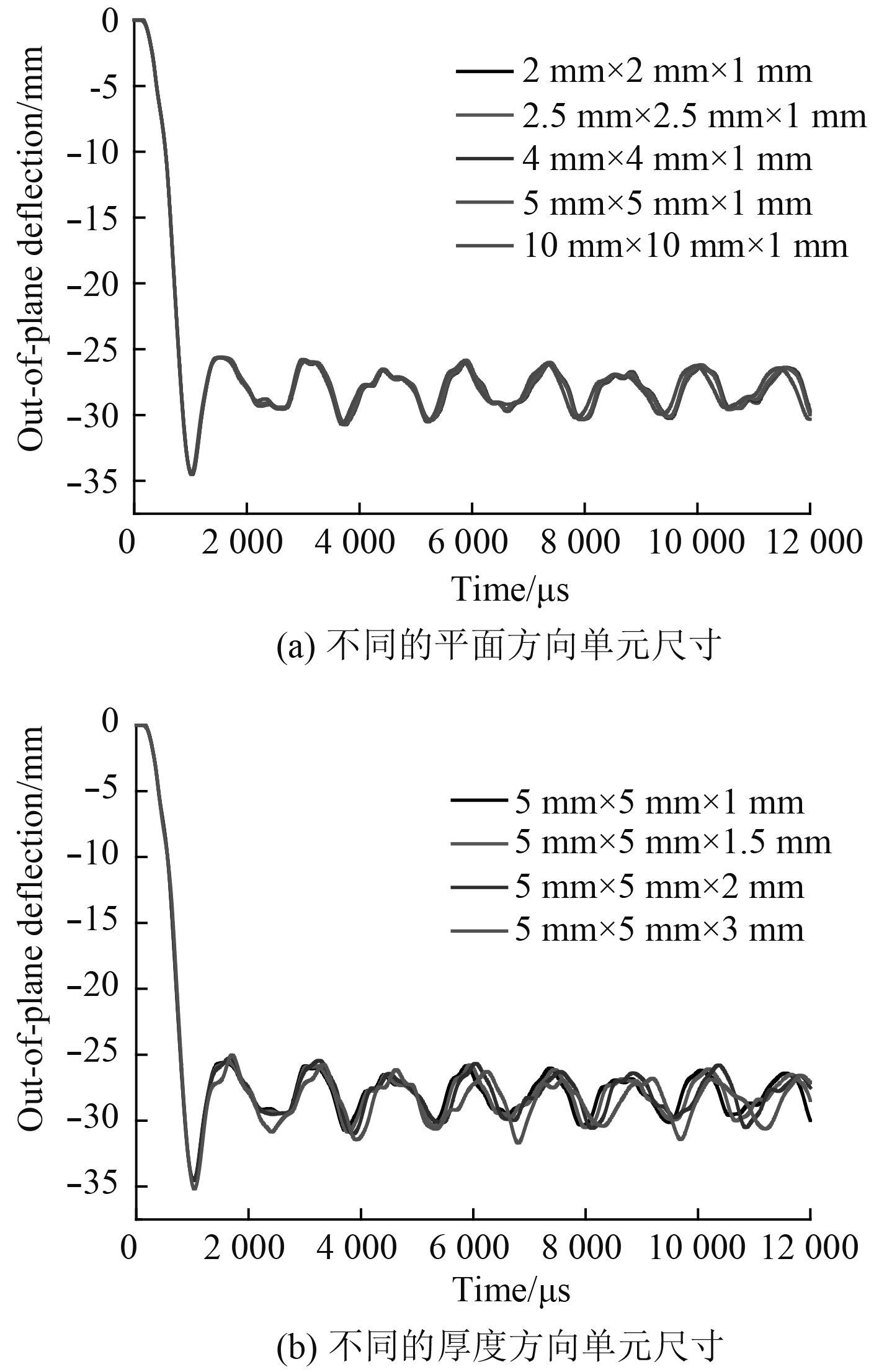图 1 不同单元尺寸条件下完好钢板最大挠度值数值计算结果 Fig. 1 Mesh sensitivity analysis showing the predicted maximum deflection (mm) of an uncorroded plate (0.5 m×0.5 m)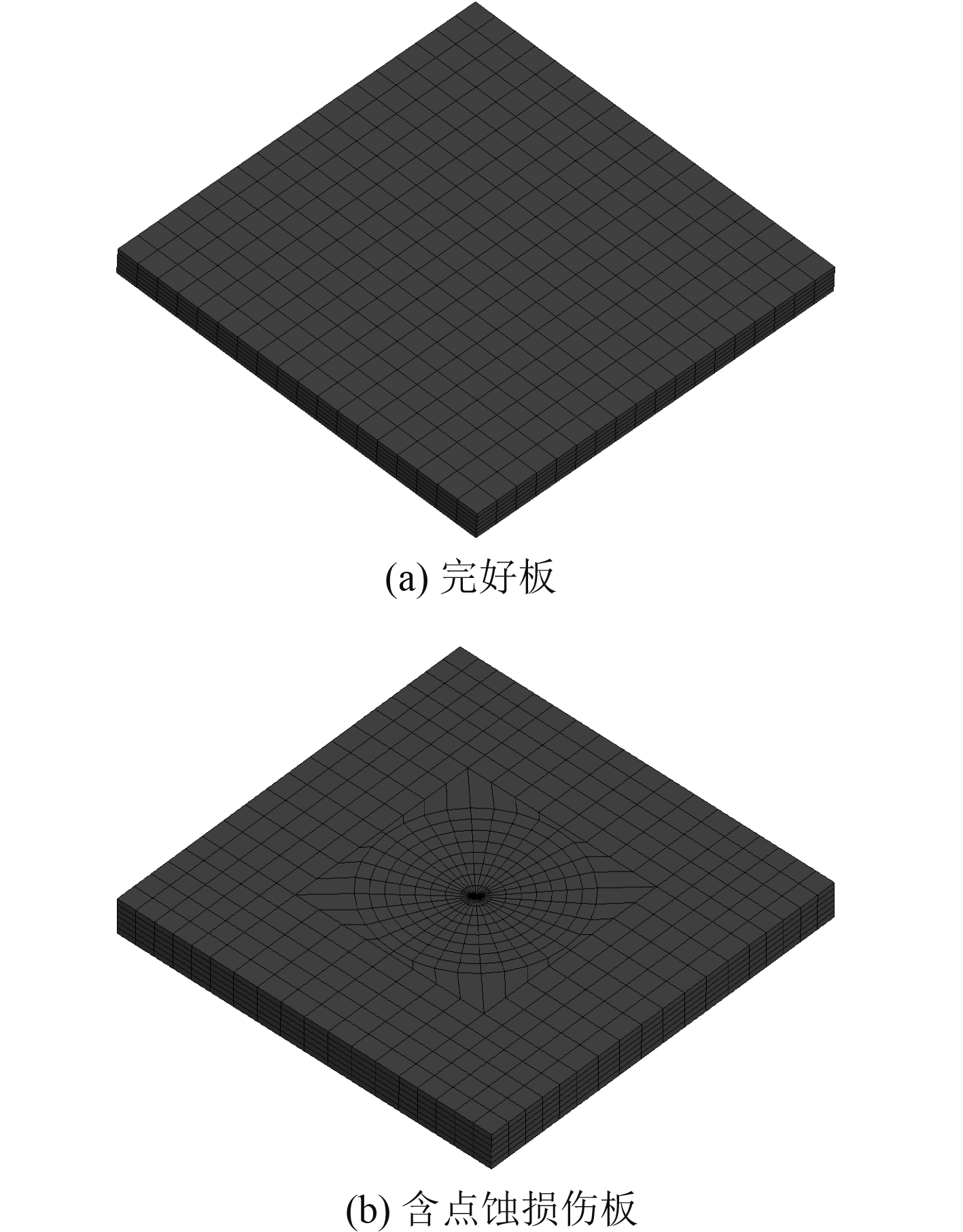图 2 数值计算模型单元划分示意图 Fig. 2 Finite element models of uncorroded and corroded plate
2.2 点蚀损伤尺寸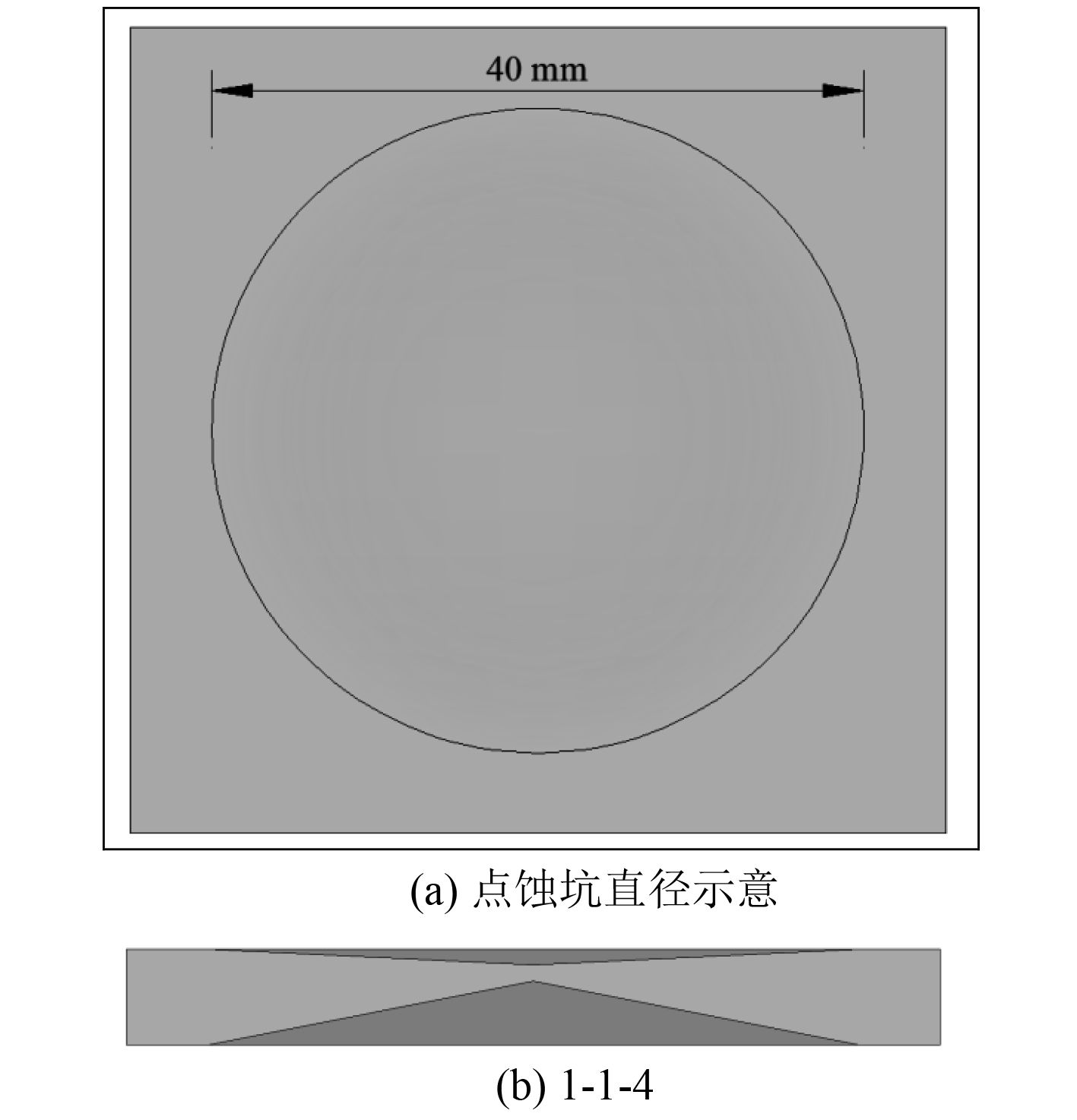图 3 典型点蚀坑的尺寸示意 Fig. 3 Geometry of representative corrosion pit
2.3 点蚀损伤分布

 $DOP = \left\{ \begin{array}{l}\frac{1}{{ab}}\sum\limits_{i = 1}^n {{S_{pi}}} \times 100\% ,\;\;\;\;\;\;\left( {{\text{单面腐蚀工况}}} \right)\text{；}\\\frac{1}{{4ab}}\sum\limits_{i = 1}^n {{S_{pi}}} \times 100\%, \;\;\;\;\left( {{\text{双面腐蚀工况}}} \right)\text{。}\end{array} \right.$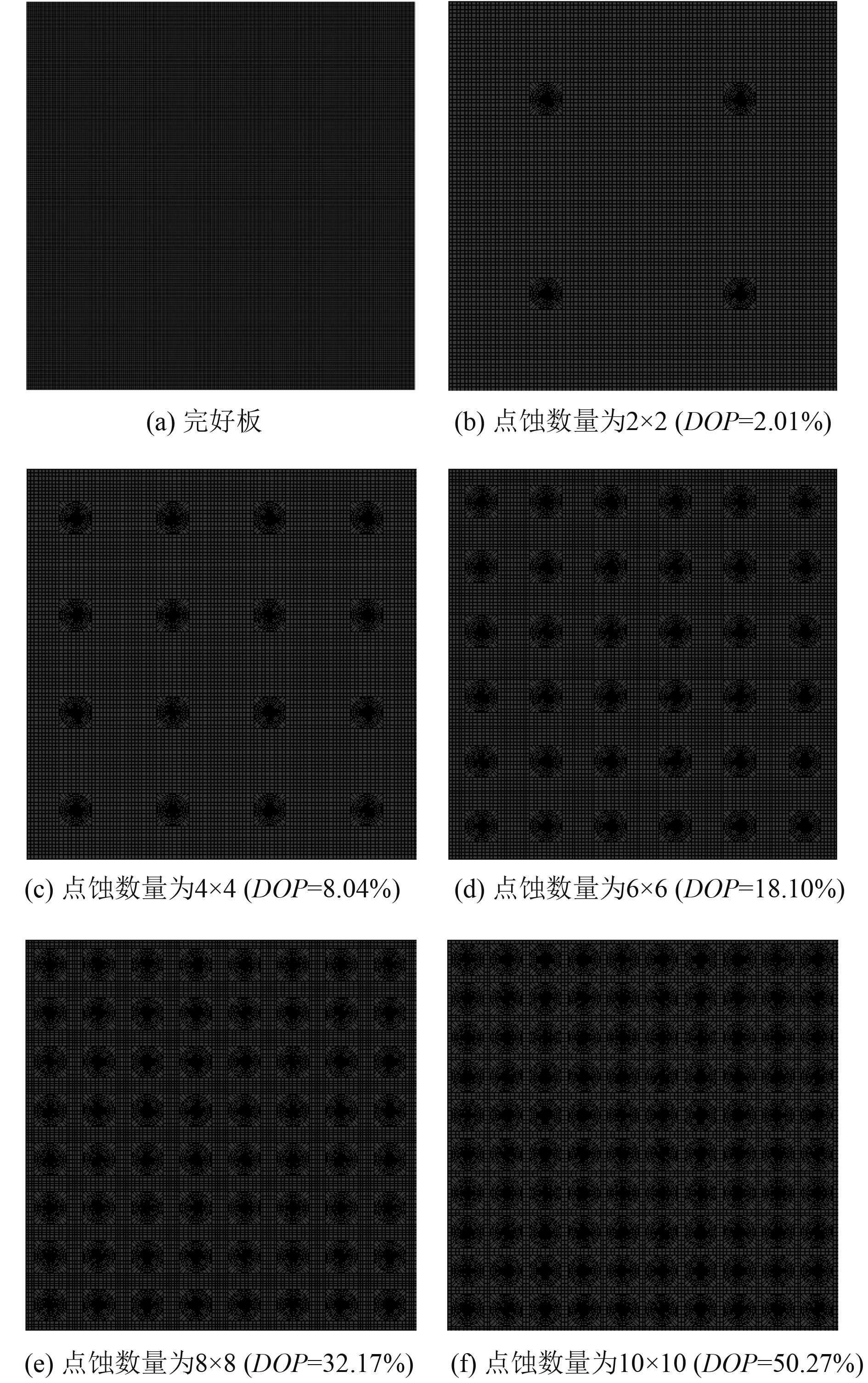图 4 点蚀坑分布示意图（0.5×0.5 m2） Fig. 4 FE models of the corroded plates with regular distributed corrosion pits (0.5×0.5 m2)
2.4 点蚀损伤板动态响应分析 2.4.1 点蚀坑深度的影响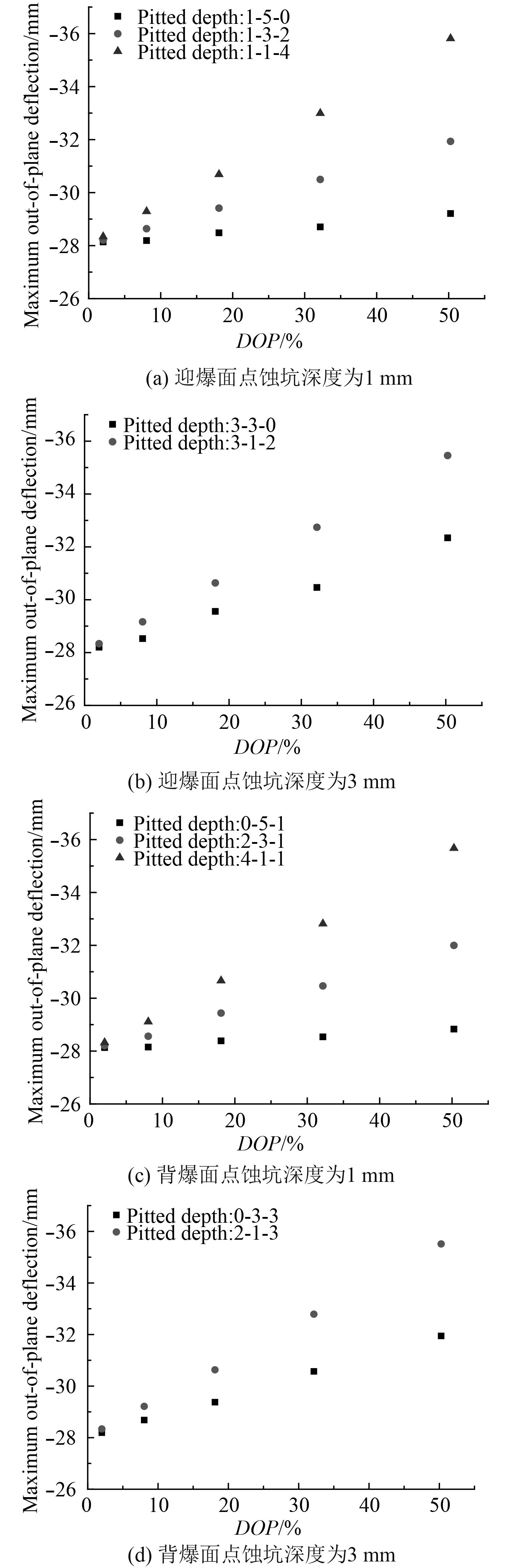图 5 点蚀深度对点蚀损伤板最大挠度值的影响数值计算结果 Fig. 5 Variation of the maximum out-of-plane deflection，as a function of DOP in plates with different corrosion depth

2.4.2 点蚀损伤分布密度的影响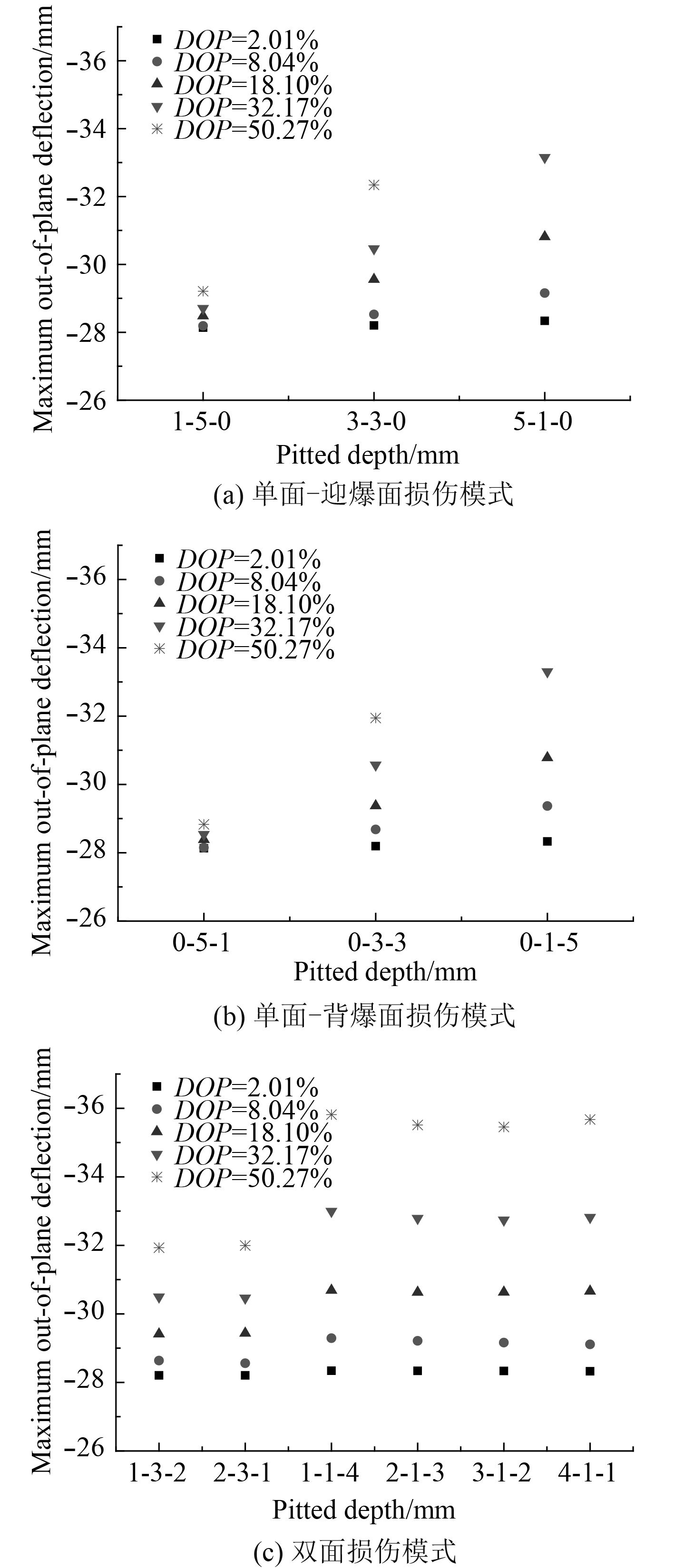图 6 点蚀坑分布密度对点蚀损伤板最大挠度值的影响数值计算结果 Fig. 6 Variation of the maximum out-of-plane deflection，as a function of corrosion patterns with different DOP in plates

2.4.3 点蚀损伤分布位置的影响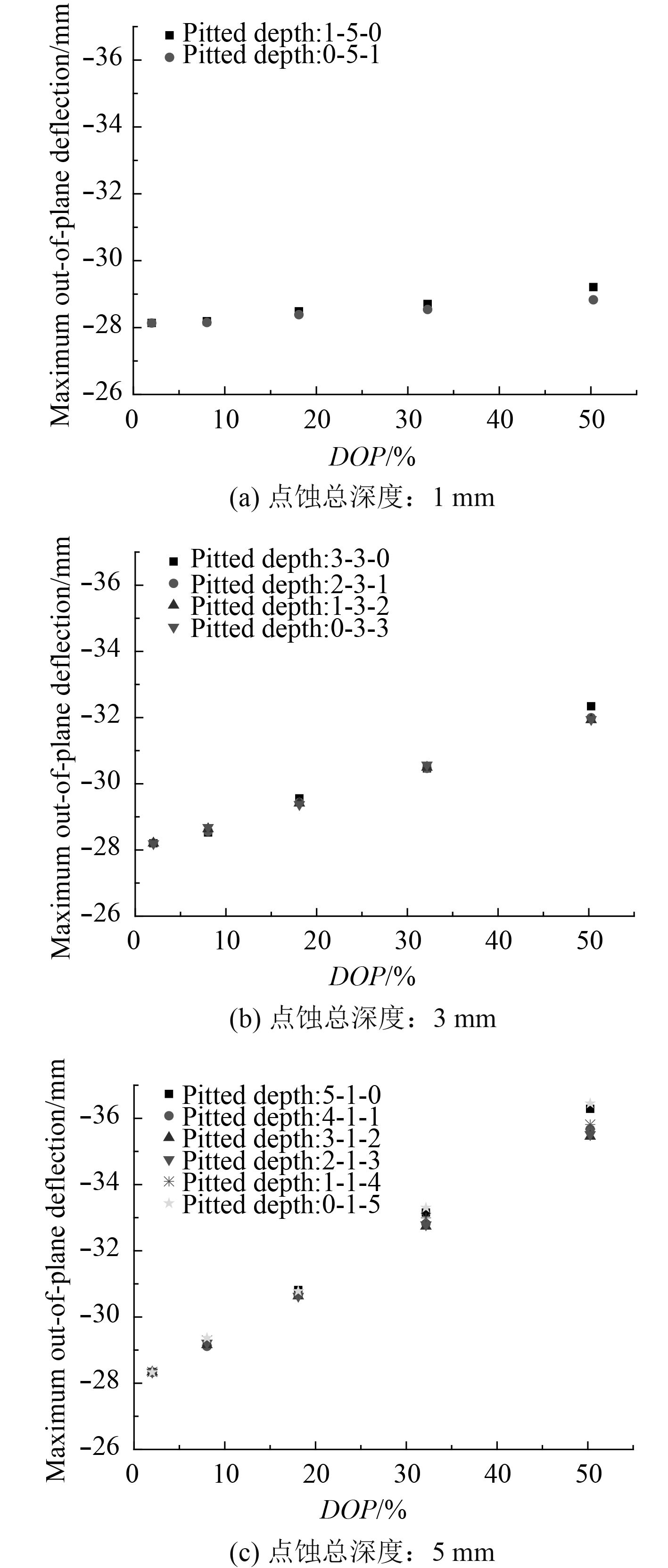图 7 点蚀损伤分布位置对点蚀损伤板最大挠度值的影响数值计算结果 Fig. 7 Variation of the maximum out-of-plane deflection，as a function of DOP in plates with different corrosion patterns

3 结　语

1）本文研究了点蚀损伤深度、点蚀损伤分布密度及点蚀损伤分布位置对钢板动态响应的影响，本文选取最大挠度值增加程度表征板的动态承载能力的降低程度，结果表明，点蚀损伤深度、点蚀损伤分布密度均对板的动态承载能力有较大影响，而点蚀损伤分布位置则影响非常小；

2）保持DOP不变的条件下，随着点蚀深度的增加，板动态承载能力随之降低，且随着DOP的增大，该趋势愈加明显；

3）在特定损伤模式下，随着DOP的增大，板动态承载能力随之增大，且随着点蚀总深度的增加，板动态承载能力增大的效果越来越显著；

4）在相同的点蚀总深度下，不同的点蚀损伤模式：单面-迎爆面点蚀损伤、单面-背爆面点蚀损伤以及双面点蚀损伤，对板动态承载能力的影响非常小，板动态承载能力对点蚀分布位置很不敏感。